# Compute numeric values in arrays with System.Linq namespaceMicrosoft .NET Framework can store values in an array which makes it easy to use in methods and properties. An array can contain any kind of object, but I most commonly use it to store numeric value.

A greate property of numeric values is that you can compute them to a different value, and use that value to derive conclusions of any kind (I might sound like a madman , but figures somehow interest me).

A simple example of computing numeric values is deriving the lowest number is a range of number or the average of all numbers. .NET has a method that can compute this easily for you, but it’s a bit hidden)

Let’s take the following range of numbers: 0,1, 2, 3, 4. The lowest number in this range is 0 and the average is 2 (0+1+2+3+4 / 5). That’s easy.

#### Example in VB.Net

In VB.Net the range of number can be placed in an array, in this example I’ll be using an array of Double . I’m looking for the lowest, highest and average of the range of numbers.

So I created a new VB.Net project, which has target framework of .NET Framework 2.0 by default, and wrote the following lines of code:

```     Dim dblResults() As Double = {0, 1, 2, 3, 4}

Debug.Print("The lowest result is : " & dblResults.Min.ToString)
Debug.Print("The highest result is : " & dblResults.Max.ToString)
Debug.Print("The average result is : " & dblResults.Average.ToString)```
###### ’…’ is not a member of  ‘System.Array’

But, like I said in the introduction, these computations seem to be unavailable as a method. They would result in the error ‘Min’ is not a member of ‘System.Array’.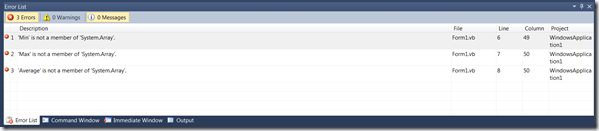###### Requirements

The methods are published as part of the namespace ‘System.Linq’, but only in .NET Framework 3.5.

So the first step is to upgrade the target framework from ‘.NET Framework 2.0’ to ‘NET Framework 3.5’. This can be achieved by opening the properties of the project and selecting the ‘Advanced Compile Settings’ in the Compile tab.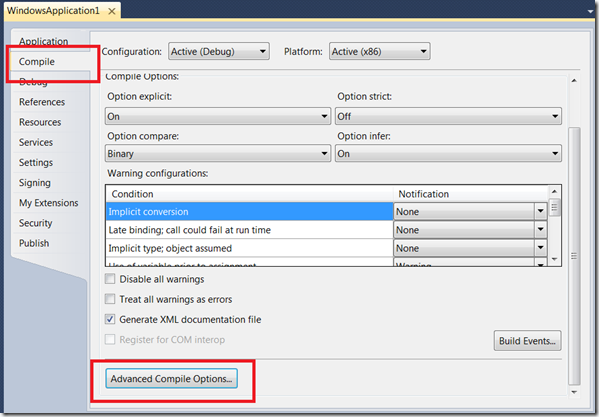In the ‘Advanced Compile Options’ select the correct .NET Framework and press OK. Confirm in the next dialog that you wan’t to upgrade the the target framework.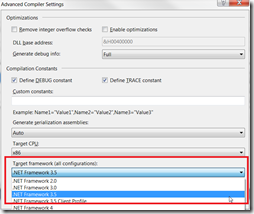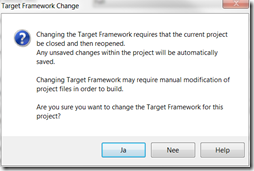###### Reference namespace ‘System.Linq’

Next step is to reference the namespace ‘System.Linq’, which can be done by clicking ‘Add’ on the tab ‘References’ and selecting the ‘System.Xml.Linq’ reference.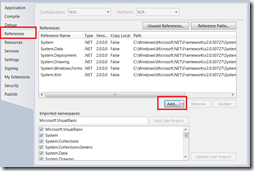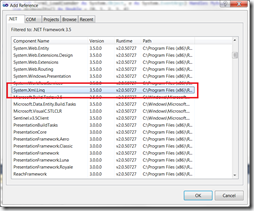###### Import System.Linq in code

Finally import the namespace in the code so the methods can be accessed in the code.

`   Imports System.Linq`
###### Running demo

Now the code is accepted and the demo application works as expected.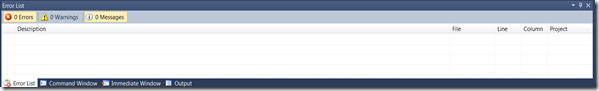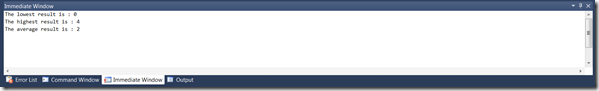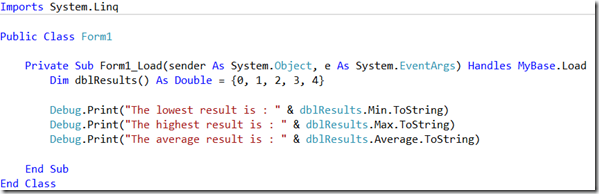###### Conclusion

Computing numeric values in arrays is available in .NET Framework but a bit hidden in the ‘System.Linq’ namespace. Although I can imagine why you want to use this methods when using Linq, I can’t imagin why you Microsoft didn’t import them in the base of .NET Framework.

Computing numeric values, as showed, are simple functions that can be created easily. But i’d rather use functions supplied by the .NET Framework and focus on my main objective. But referencing a namespace, like System.Linq, does have an impact on the baseline of the application (and therefore performance footprint).

Ingmar Verheij

Deze site gebruikt Akismet om spam te verminderen. Bekijk hoe je reactie-gegevens worden verwerkt.